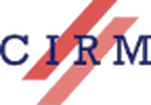Independence of normal words   (pdf)

Random numbers as probabilities of machine behaviour   (pdf)

The Computational Power of Sets of Random Strings   (pdf)

Zero sets  and local time of algorithmically random Brownian motion   (pdf)

Carleson’s Theorem and Schnorr randomness   (pdf)

On the entropy algorithmics of computable dynamical systems   (pdf)

Borel isomorphism and Computability   (pdf)

A derivation on the field of d.c.e.reals   (pdf)

On totally omega-c.e. degrees and complex left-c.e.reals   (pdf)

The Gamma question   (pdf)

Randomness connecting to set theory and to reverse mathematics   (pdf)

Gambling Against Some Odds   (pdf)

On Centauric Subshifts   (pdf)

Ultralimits and computability   (pdf)

On Block Pumpable Languages   (pdf)

Seas of squares   (pdf)

On higher Friedman’s conjecture   (pdf)